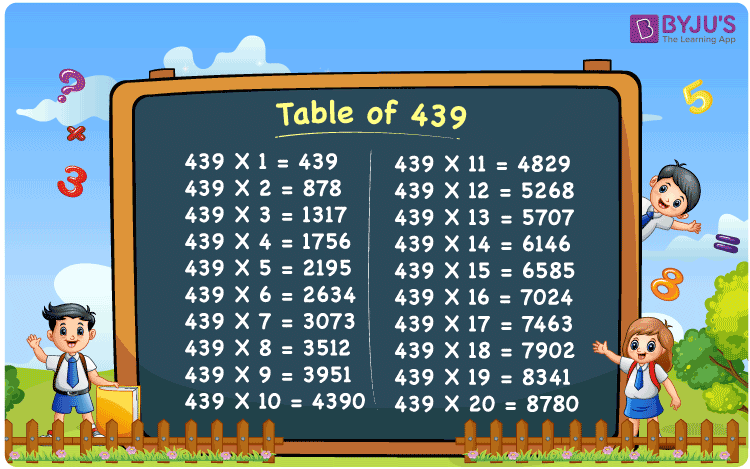Checkout JEE MAINS 2022 Question Paper Analysis : Checkout JEE MAINS 2022 Question Paper Analysis :

# Table of 439

The Table of 439 is the best reference guide for the students among the numerous materials available on the internet today. Each and every minute concept is explained in a comprehensive manner, so that students get a clear knowledge of the concepts in their lower classes itself. For this reason, the experienced faculty has designed the table of 439 in the most creative way possible to help students learn the multiplication tables thoroughly. By just clicking on the PDF download link given here, the multiplication table of 439 can be accessed by the students absolutely free of cost.

## Table of 439 Chart## What is the 439 Times Table?

Learning the multiplication table of 439 is very essential for the students in order to get a good grasp of the basic concepts of Mathematics. We all know that Multiplication, Division, Addition and Subtraction are the fundamental operations of the subject and one who masters all these will be able to face the difficult questions that might appear in the final exams.

 439 × 1 = 439 439 439 × 2 = 878 439 + 439 = 878 439 × 3 = 1317 439 + 439 + 439 = 1317 439 × 4 = 1756 439 + 439 + 439 + 439 = 1756 439 × 5 = 2195 439 + 439 + 439 + 439 + 439 = 2195 439 × 6 = 2634 439 + 439 + 439 + 439 + 439 + 439 = 2634 439 × 7 = 3073 439 + 439 + 439 + 439 + 439 + 439 + 439 = 3073 439 × 8 = 3512 439 + 439 + 439 + 439 + 439 + 439 + 439 + 439 = 3512 439 × 9 = 3951 439 + 439 + 439 + 439 + 439 + 439 + 439 + 439 + 439 = 3951 439 × 10 = 4390 439 + 439 + 439 + 439 + 439 + 439 + 439 + 439 + 439 + 439 = 4390

## Multiplication Table of 439

The Table of 439 basically provides a clear idea about the multiplication of 439 with numbers till 20 in the form of a chart. Students who do not have a good knowledge of the multiplication tables can use the PDF provided at BYJU’S, as a reference. Each and every step is calculated accurately by the subject experts with the aim of providing the students with the best reference material possible.

 439 × 1 = 439 439 × 2 = 878 439 × 3 = 1317 439 × 4 = 1756 439 × 5 = 2195 439 × 6 = 2634 439 × 7 = 3073 439 × 8 = 3512 439 × 9 = 3951 439 × 10 = 4390 439 × 11 = 4829 439 × 12 = 5268 439 × 13 = 5707 439 × 14 = 6146 439 × 15 = 6585 439 × 16 = 7024 439 × 17 = 7463 439 × 18 = 7902 439 × 19 = 8341 439 × 20 = 8780

## Solved Example on the Table of 439

Q.1: 10 litres of milk were supplied from the dairy in a day. How many litres of milk will be supplied in 439 days?

Solution: Given,

Number of litres of milk supplied in a day = 10

In 439 days number of litres of milk that will be supplied = 439 x 10

= 4390

## Frequently Asked Questions on the Table of 439

### Find the value of 439 multiplied by 12.

Using the multiplication table of 439, 439 multiplied by 12 is 439 x 12 = 5268.

### With which number should we multiply 439 to get 7024?

With the help of the table of 439, 439 x 16 = 7024. Hence, 439 should be multiplied by 16 to get 7024.

### What is 439 times 16 plus 2 minus 13?

In the table of 439, 439 times 16 plus 2 minus 13 can be written as 439 x (16 + 2 – 13) = 439 x 5 = 2195